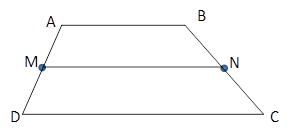Chapter 4.4, Problem 13EElementary Geometry For College St...

7th Edition
Alexander + 2 others
ISBN: 9781337614085

Solutions

Chapter
SectionElementary Geometry For College St...

7th Edition
Alexander + 2 others
ISBN: 9781337614085
Textbook Problem

In Exercises 11 to 16, the drawing shows trapezoid ABCD with A B ¯ | | D C ¯ ; also, M and N are midpoints of A D ¯ and B C ¯ , respectively.Exercises 11-16Given: A B = 8.2   a n d   M N = 9.5 Find: D C

To determine

To Find:

DC of trapezoid ABCD.

Explanation

Consider the following trapezoid ABCD.

Given:

AB=8.2 and MN=9.5

In the given trapezoid, AB¯||DC¯.

M is the midpoint of the side AD¯ and N is the midpoint of the side BC¯

Still sussing out bartleby?

Check out a sample textbook solution.

See a sample solution

The Solution to Your Study Problems

Bartleby provides explanations to thousands of textbook problems written by our experts, many with advanced degrees!

Get Started

Solve the equations in Exercises 126. (x2+1)x+1(x+1)3=0

Finite Mathematics and Applied Calculus (MindTap Course List)

In Exercises 2340, find the indicated limit. 40. limx3xx2+72x2x+3

Applied Calculus for the Managerial, Life, and Social Sciences: A Brief Approach

Evaluate the integral 39. 01/2xe2x(1+2x)2dx

Single Variable Calculus: Early Transcendentals

The graph at the right is the direction field for: a) y = x y b) y = xy c) y = x + y d) y = xy

Study Guide for Stewart's Single Variable Calculus: Early Transcendentals, 8th